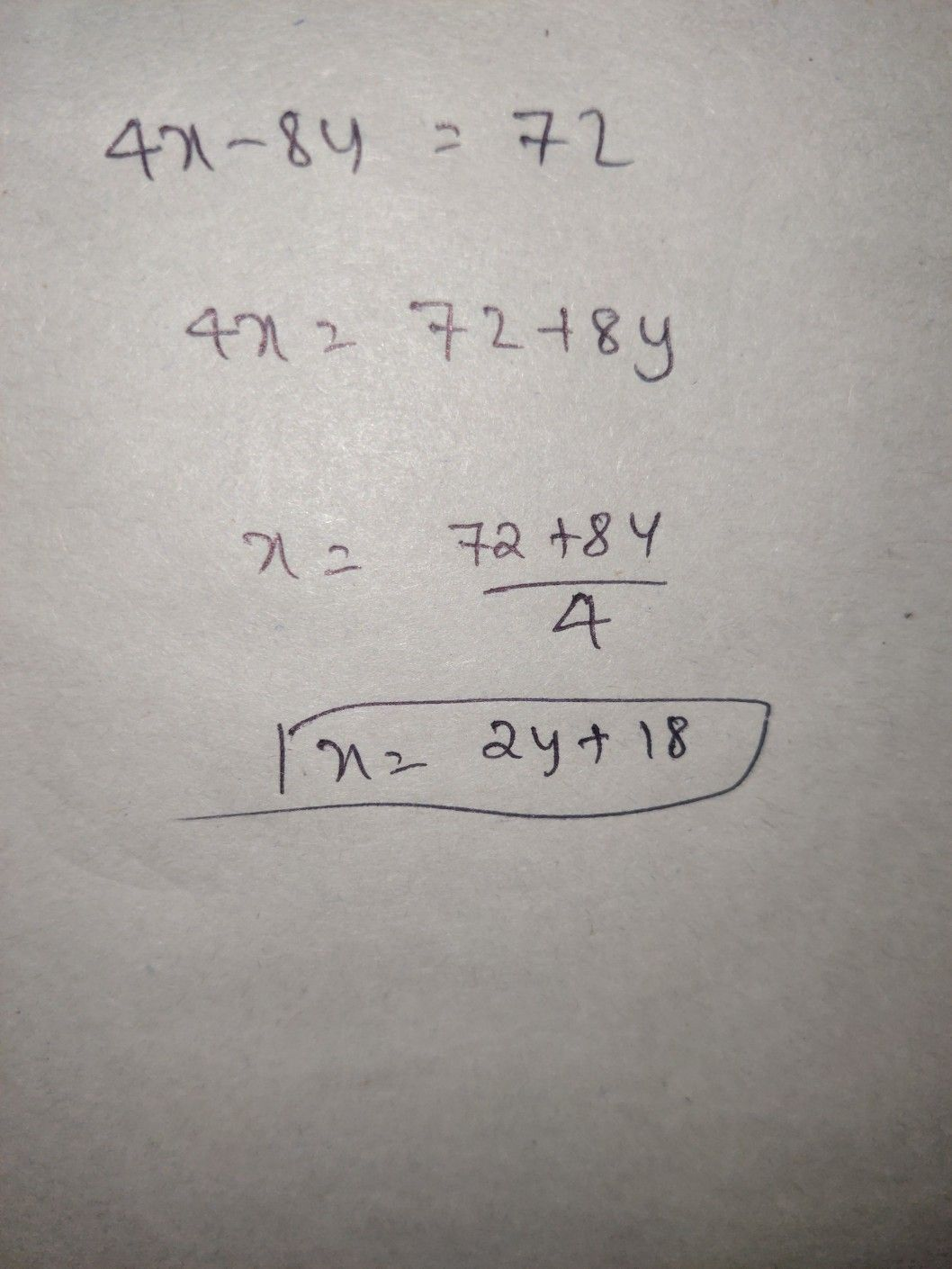Symbol
Problem$4x$ $=$ $3$ $\pi$ $=32$